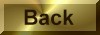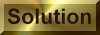Practice Problem 5

CalculateH° andS° for the following reaction and decide in which direction each of these factors will drive the reaction.

N2(g) + 3 H2(g)2 NH3(g)Ho = -92.22 kJSo = -198.75 J/K